# Waring problem

(diff) ← Older revision | Latest revision (diff) | Newer revision → (diff)

A problem in number theory formulated in 1770 by E. Waring in the following form: Any natural number is a sum of 4 squares, of 9 cubes and of 19 fourth-powers. In other words, for allthere exists a, depending only on, such that every natural number is the sum of-th powers of non-negative integers. D. Hilbert in 1909 was the first to give a general solution of Waring's problem with a very rough estimate of the value ofas a function of; this is why the problem is sometimes known as the Hilbert–Waring problem. Let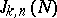be the number of solutions of the equation(1)

in non-negative integers. Hilbert's theorem then states that there exists a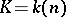for which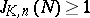for any. G.H. Hardy and J.E. Littlewood, who applied the circle method to the Waring problem, demonstrated in 1928 that forthe value ofis given by an asymptotic formula of the type(2)

where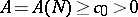, while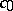and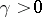are constants. Consequently, if, equation (1) has a solution. This result gave rise to three problems: Determine the order of the three quantities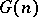,,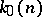which are the smallest integers for which: a) equation (1) is solvable for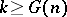and; b) equation (1) is solvable for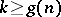and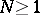; or c) the asymptotic formula (2) applies toif.

a) It is known that. It was proved in 1934 by I.M. Vinogradov, using his own method, that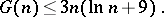Moreover, many results are available concerningfor small values of:(H. Davenport, 1939);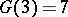(Yu.V. Linnik, 1942).

b) It was shown in 1936 by L. Dickson and S. Pillai, who also used the Vinogradov method, that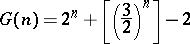for all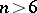for which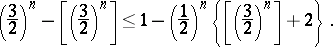The last condition was demonstrated in 1957 by K. Mahler for all sufficiently large.

c) The best result of all must be credited to Vinogradov, who showed thatAn elementary proof of Waring's problem was given in 1942 by Yu.V. Linnik. There exist many different generalizations of Waring's problem (the variables run through a certain subset of the set of natural numbers; the numberis represented by polynomials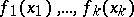rather than by monomials; equation (1) is replaced by a congruence, etc.).

The special importance of Waring's problem consists in the fact that in trying to solve it, powerful methods in analytic number theory had to be created.

How to Cite This Entry:
Waring problem. Encyclopedia of Mathematics. URL: http://encyclopediaofmath.org/index.php?title=Waring_problem&oldid=15347
This article was adapted from an original article by A.A. Karatsuba (originator), which appeared in Encyclopedia of Mathematics - ISBN 1402006098. See original article